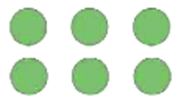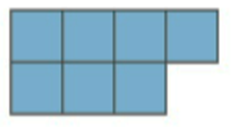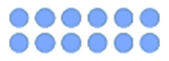Mathematics
Easy

Question

# Choose the image which represents the odd number.

##Hint:

## The correct answer is:### Here, we have to select the figure which represents the odd number.Number of circles = 6 and 12, Number of squares = 7 and Number of stars = 8 .Clearly, only 7 is not divisible by 2 i.e 7 is odd.Hence, the correct option is (b)

A number whose one's place digit is 1,3,5,7,9 is not divisible by 2 .

### Related Questions to study#### With Turito Foundation.#### Get an Expert Advice From Turito.